# GSoC Week 5: Adventures in Profiling

Posted on June 20, 2014

This week I've been working on little fixes. Improving the docstrings, a couple interface improvements, finalizing some design decisions. The plan is to finish up this work next week, and then move on to the code generation portion of the project. In it's current state, the linearizer can handle KanesMethod and LagrangesMethod with ease. I'll probably spend some time hacking away at the matrix_to_linearizer function as well, but that's not priority.

The rest of the week was spent profiling and doing some basic optimizations. Being a mechanical engineer, I haven't spent much time learning about numerical methods (although, I am taking this MIT OCW course this summer). As such, my natural way of solving the system

$$A x = B$$

where $A$, $B$, and $x$ are matrices, is to take the inverse of $A$, and multiply it by $B$:

$$x = A^{-1} B$$

Turns out, this is horribly inefficient. But what is the best way? I had to do some profiling.

# Creating Benchmark Matrices¶

For most systems of the form $A x = B$ in sympy.physics.mechanics, $A$ is a symmetric matrix, and $B$ is a column vector. To do proper benchmarks, I'd need to create random matrices of this form. Looking at some example equations of motion, it can be seen that these mostly consisted of products and sums of terms composed of symbols, trigonometric functions, power functions, sqrt, and inv. After some time, I was able to create a couple functions to create these matrices in a composable way:

In :
# Below are some functions to create random matrices of varying sizes.
# These will be used in the benchmarks.

from random import sample, randint
from sympy import Matrix, symbols, sin, cos, tan, sqrt, zeros, \
Symbol, diag, prod

# Define some operations
pow2 = lambda x: pow(x, 2)
pow3 = lambda x: pow(x, 3)
pow4 = lambda x: pow(x, 4)
inv = lambda x: 1/x

# Defaults
# OPS is a list of common operations in sympy.physics.mechanics
OPS = [sin, cos, tan, pow2, pow3, pow4, sqrt, inv]
# SYMS is a list of symbols that could be found in a matrix
SYMS = symbols('a, b, c, d, e, f, g, h, i, j')

def sum_or_prod(vec):
""" Return either the sum or product of a vector """
func = sample([sum, prod], 1)
return func(vec)

def randterms(n, max_terms, ops=OPS, syms=SYMS):
""" Creates a list of random terms of size n. Each cell is composed of
0 to max_terms, composed of randomly sampled functions from ops, and
symbols from syms """
ntermlist = [randint(1, max_terms) for i in range(n)]
pick = lambda vec, nlist: [[vec[i] for i in
sample(range(len(vec)), n)] for n in nlist]
rops = pick(ops, ntermlist)
rsyms = pick(syms, ntermlist)
terms = [zip(*opsym) for opsym in zip(rops, rsyms)]
return [sum_or_prod(op(s) for (op, s) in term) for term in terms]

def randmat(m, n, max_terms, ops=OPS, syms=SYMS):
""" Creates a random matrix of size (m,n). Each cell is composed of
1 to max_terms, composed of randomly sampled functions from ops, and
symbols from syms """
return Matrix(randterms(m*n, max_terms, ops, syms)).reshape(m, n)

def randuppertriag(n, max_terms, ops=OPS, syms=SYMS):
""" Creates a random upper triangular matrix of size (n, n). Each cell is
composed of 1 to max_terms, composed of randomly sampled functions from
ops, and symbols from syms """
nupper = sum(range(n))
terms = randterms(nupper, max_terms, ops, syms)
t = 0
rows = []
for i in range(1, n):
rows.append(zeros(1, i).row_join(Matrix(terms[t:t+n-i]).T))
t += n-i
rows.append(zeros(1, n))
return Matrix(rows)

def symrandmat(n, max_terms, ops=OPS, syms=SYMS):
""" Creates a random symmetric matrix of size (n, n). Each cell is
composed of 1 to max_terms, composed of randomly sampled functions from
ops, and symbols from syms """
upper = randuppertriag(n, max_terms, ops, syms)
lower = upper.T
D = diag(*randterms(n, max_terms, ops, syms))
return upper + D + lower

In :
# 3x3 Random Upper Triangular Matrix with max number of terms 2
randuppertriag(3, 2)

Out:
Matrix([
[0, cos(e),      tan(g)],
[0,      0, f**4*cos(j)],
[0,      0,           0]])
In :
# 3x3 Random Matrix with max number of terms 2
randmat(3, 3, 2)

Out:
Matrix([
[   b**4, j**2 + tan(c),           d**4],
[    1/d,        cos(f), c**2 + sqrt(d)],
[sqrt(a),        cos(g),           j**4]])
In :
# 3x3 Symmetric matrix with max number of terms 2
symrandmat(3, 2)

Out:
Matrix([
[          cos(a), sqrt(g) + sin(b),        tan(f)],
[sqrt(g) + sin(b),   sqrt(i)*cos(j),           1/b],
[          tan(f),              1/b, cos(f)*tan(c)]])

# Solution Methods¶

There are 4 different methods we'll be testing:

1. LUsolve: Solve the problem with LU decomposition
2. LDLsolve: For symmetric matrices, solve with LDL decomposition
3. cholesky_solve: For symmetric matrices, solve with cholesky decomposition
4. _mat_inv_mul: Solve using LDL decomposition, and an intermediate substitution dictionary. This is what mechanics is currently using.

After doing some reading up on these, LDLsolve and cholesky_solve should be the fastest, as the $A$ matrix is symmetric. _mat_inv_mul also uses LDL decomposition, but it has the overhead of substitution. However, for larger matrices this may yield benefits, as the LDL decomposition is performed only on a matrix of single symbols.

In :
# This is what sympy.physics.mechanics is currently using.
# Copied into this file, to show what it's doing.
def _mat_inv_mul(A, B):
"""
Computes A^-1 * B symbolically w/ substitution, where B is not
necessarily a vector, but can be a matrix.

"""

r1, c1 = A.shape
r2, c2 = B.shape
temp1 = Matrix(r1, c1, lambda i, j: Symbol('x' + str(j) + str(r1 * i)))
temp2 = Matrix(r2, c2, lambda i, j: Symbol('y' + str(j) + str(r2 * i)))
for i in range(len(temp1)):
if A[i] == 0:
temp1[i] = 0
for i in range(len(temp2)):
if B[i] == 0:
temp2[i] = 0
temp3 = []
for i in range(c2):
temp3.append(temp1.LDLsolve(temp2[:, i]))
temp3 = Matrix([i.T for i in temp3]).T
return temp3.subs(dict(list(zip(temp1, A)))).subs(dict(list(zip(temp2, B))))


# Benchmark: Constant Matrix Complexity, Varying n¶

Here we check increasing matrix dimensions, with a constant complexity of each cell (max_terms = 3). Only running up to n=6, as computation got excessive after that.

In :
from timeit import repeat

# Run the benchmark for varying values of n, with max_terms = 3
timestmt = lambda stmt, setup, n, r: min(repeat(stmt, setup=setup, repeat=r, number=n))/n
ns = range(2, 7)
lu_t = []
ldl_t = []
chol_t = []
matinv_t = []
for n in ns:
A = symrandmat(n, 3)
B = randmat(n, 1, 3)
lu_t.append(timestmt('A.LUsolve(B)', 'from __main__ import A, B', 1, 3))
ldl_t.append(timestmt('A.LDLsolve(B)', 'from __main__ import A, B', 1, 3))
chol_t.append(timestmt('A.cholesky_solve(B)', 'from __main__ import A, B', 1, 3))
matinv_t.append(timestmt('_mat_inv_mul(A, B)',
'from __main__ import A, B, _mat_inv_mul', 1, 1))

In :
# Plot the results
import matplotlib.pyplot as plt
% matplotlib inline

plt.figure()
plt.plot(ns, lu_t, label='A.LUsolve(B)')
plt.plot(ns, ldl_t, label='A.LDLsolve(B)')
plt.plot(ns, chol_t, label='A.cholesky_solve(B)')
plt.plot(ns, matinv_t, label='mat_inv_mul(A, B)')
plt.xlabel('Matrix Dimension')
plt.ylabel('Time (s)')
plt.title('Timings for Solution to A*x = b, Varying Dimensions')
plt.legend(loc=2)
plt.figure()
plt.plot(ns, lu_t, label='A.LUsolve(B)')
plt.plot(ns, ldl_t, label='A.LDLsolve(B)')
plt.plot(ns, chol_t, label='A.cholesky_solve(B)')
plt.xlabel('Matrix Dimension')
plt.ylabel('Time (s)')
plt.title('Timings for Solution to A*x = b,  Varying Dimensions')
plt.legend(loc=2)
plt.show()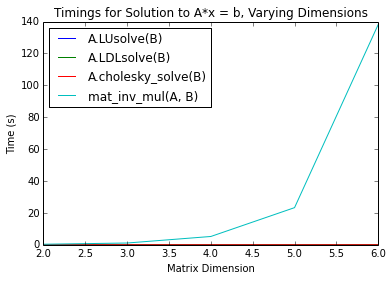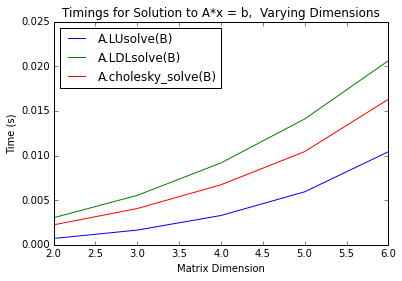Observing the above plots it can be seen that _mat_inv_mul is several orders of magnitude slower than the other three methods, and increases in time at a faster rate. As the underlying algorithm is the same as LDLsolve, this indicates that the subs operations are slow, and of larger complexity than LDLsolve. While the other three methods are all close, LUsolve is the fastest. This is interesting, because for purely numeric computation (i.e. floating point only), LDLsolve and cholesky_solve should be faster for symmetric matrices.

# Benchmark: Varying Matrix Complexity, Constant n¶

Here we vary the complexity of the matrix (how many terms in each cell), but keep the matrix size constant. I picked n = 4, because it was big enough to take some time, but not too big to take forever. I'd expect to see the computation time increase with complexity, but not as rapidly as it did with matrix dimension.

In :
# Run the benchmark for varying values of max_terms, with n = 4
ms = range(2, 9)
lu_t = []
ldl_t = []
chol_t = []
matinv_t = []
for m in ms:
A = symrandmat(4, m)
B = randmat(4, 1, m)
lu_t.append(timestmt('A.LUsolve(B)', 'from __main__ import A, B', 1, 3))
ldl_t.append(timestmt('A.LDLsolve(B)', 'from __main__ import A, B', 1, 3))
chol_t.append(timestmt('A.cholesky_solve(B)', 'from __main__ import A, B', 1, 3))
matinv_t.append(timestmt('_mat_inv_mul(A, B)',
'from __main__ import A, B, _mat_inv_mul', 1, 1))

In :
# Plot the results
plt.figure()
plt.plot(ms, lu_t, label='A.LUsolve(B)')
plt.plot(ms, ldl_t, label='A.LDLsolve(B)')
plt.plot(ms, chol_t, label='A.cholesky_solve(B)')
plt.plot(ms, matinv_t, label='mat_inv_mul(A, B)')
plt.xlabel('Matrix Complexity')
plt.ylabel('Time (s)')
plt.title('Timings for Solution to A*x = b, Varying Complexity')
plt.legend(loc=2)
plt.figure()
plt.plot(ms, lu_t, label='A.LUsolve(B)')
plt.plot(ms, ldl_t, label='A.LDLsolve(B)')
plt.plot(ms, chol_t, label='A.cholesky_solve(B)')
plt.xlabel('Matrix Complexity')
plt.ylabel('Time (s)')
plt.title('Timings for Solution to A*x = b, Varying Complexity')
plt.legend(loc=2)
plt.show()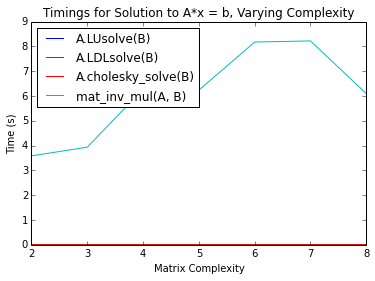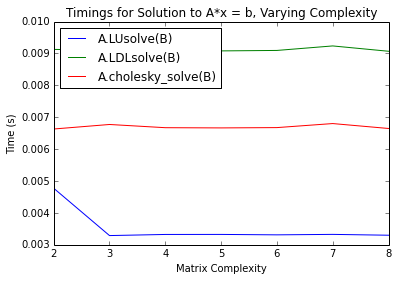Interestingly, it seems that LUsolve, LDLsolve, and cholesky_solve don't increase in time with complexity, while _mat_inv_mul does. This must be due to the intermediate substitution step.

# Benchmark: Turning Matrix Term Complexity up to 11¶

Using the matrices composed by the above methods, all cells are either a sum of terms, or a product. This is close to how the matrices we need to solve look, but it's not a perfect representation. Let's try with a really ugly matrix. We can use the fact that for any matrix $A$, $A A^T$ is a symmetric matrix.

In :
temp = prod([randmat(4, 4, 2) for i in range(2)])
A = temp*temp.T
B = randmat(4,1,6)

In :
A

Out:
Matrix([
[                                                                                                                                                                                                                                                                                                   (h**(9/2) + (sqrt(a) + cos(i))*(c**4 + tan(f)) + sin(i)*cos(c) + sin(f)*sin(g)/c)**2 + (c*sin(g) + (sqrt(a) + cos(i))*tan(g) + sin(i)*cos(c) + sqrt(h)*tan(a)/e)**2 + (sqrt(h)*(b**4 + sqrt(h)) + sqrt(i)*(sqrt(a) + cos(i)) + sin(a)*cos(c) + i**2*sin(g)*sin(j)/c)**2 + (b**2*e**4*cos(c) + d**3*(sqrt(a) + cos(i)) + g**2*sqrt(h) + (g**4 + cos(f))*sin(g)/c)**2, (h**(9/2) + (sqrt(a) + cos(i))*(c**4 + tan(f)) + sin(i)*cos(c) + sin(f)*sin(g)/c)*(d**3*sin(i) + j**3*sin(f) + h**4/f + h**2*(c**4 + tan(f))/f) + (c*sin(g) + (sqrt(a) + cos(i))*tan(g) + sin(i)*cos(c) + sqrt(h)*tan(a)/e)*(c**2*j**3 + d**3*sin(i) + h**2*tan(g)/f + tan(a)/(e*f)) + (d**3*sin(a) + i**2*j**3*sin(j) + h**2*sqrt(i)/f + (b**4 + sqrt(h))/f)*(sqrt(h)*(b**4 + sqrt(h)) + sqrt(i)*(sqrt(a) + cos(i)) + sin(a)*cos(c) + i**2*sin(g)*sin(j)/c) + (b**2*d**3*e**4 + d**3*h**2/f + j**3*(g**4 + cos(f)) + g**2/f)*(b**2*e**4*cos(c) + d**3*(sqrt(a) + cos(i)) + g**2*sqrt(h) + (g**4 + cos(f))*sin(g)/c), (h**(9/2) + (sqrt(a) + cos(i))*(c**4 + tan(f)) + sin(i)*cos(c) + sin(f)*sin(g)/c)*(c**2*sin(f)/h + h**4*(sin(a) + cos(b)) + (c**4 + tan(f))/c + sin(i)/c) + (c*sin(g) + (sqrt(a) + cos(i))*tan(g) + sin(i)*cos(c) + sqrt(h)*tan(a)/e)*(c**4/h + (sin(a) + cos(b))*tan(a)/e + sin(i)/c + tan(g)/c) + (sqrt(h)*(b**4 + sqrt(h)) + sqrt(i)*(sqrt(a) + cos(i)) + sin(a)*cos(c) + i**2*sin(g)*sin(j)/c)*(c**2*i**2*sin(j)/h + (b**4 + sqrt(h))*(sin(a) + cos(b)) + sqrt(i)/c + sin(a)/c) + (b**2*e**4/c + c**2*(g**4 + cos(f))/h + g**2*(sin(a) + cos(b)) + d**3/c)*(b**2*e**4*cos(c) + d**3*(sqrt(a) + cos(i)) + g**2*sqrt(h) + (g**4 + cos(f))*sin(g)/c), (h**(9/2) + (sqrt(a) + cos(i))*(c**4 + tan(f)) + sin(i)*cos(c) + sin(f)*sin(g)/c)*(sqrt(b)*g**2*sin(f) + sqrt(e)*sin(i) + f**2*h**4 + (c**4 + tan(f))*tan(i)) + (c*sin(g) + (sqrt(a) + cos(i))*tan(g) + sin(i)*cos(c) + sqrt(h)*tan(a)/e)*(sqrt(b)*c**2*g**2 + sqrt(e)*sin(i) + tan(g)*tan(i) + f**2*tan(a)/e) + (sqrt(h)*(b**4 + sqrt(h)) + sqrt(i)*(sqrt(a) + cos(i)) + sin(a)*cos(c) + i**2*sin(g)*sin(j)/c)*(sqrt(b)*g**2*i**2*sin(j) + sqrt(e)*sin(a) + f**2*(b**4 + sqrt(h)) + sqrt(i)*tan(i)) + (sqrt(b)*g**2*(g**4 + cos(f)) + b**2*e**(9/2) + d**3*tan(i) + f**2*g**2)*(b**2*e**4*cos(c) + d**3*(sqrt(a) + cos(i)) + g**2*sqrt(h) + (g**4 + cos(f))*sin(g)/c)],
[                                                  (h**(9/2) + (sqrt(a) + cos(i))*(c**4 + tan(f)) + sin(i)*cos(c) + sin(f)*sin(g)/c)*(d**3*sin(i) + j**3*sin(f) + h**4/f + h**2*(c**4 + tan(f))/f) + (c*sin(g) + (sqrt(a) + cos(i))*tan(g) + sin(i)*cos(c) + sqrt(h)*tan(a)/e)*(c**2*j**3 + d**3*sin(i) + h**2*tan(g)/f + tan(a)/(e*f)) + (d**3*sin(a) + i**2*j**3*sin(j) + h**2*sqrt(i)/f + (b**4 + sqrt(h))/f)*(sqrt(h)*(b**4 + sqrt(h)) + sqrt(i)*(sqrt(a) + cos(i)) + sin(a)*cos(c) + i**2*sin(g)*sin(j)/c) + (b**2*d**3*e**4 + d**3*h**2/f + j**3*(g**4 + cos(f)) + g**2/f)*(b**2*e**4*cos(c) + d**3*(sqrt(a) + cos(i)) + g**2*sqrt(h) + (g**4 + cos(f))*sin(g)/c),                                                                                                                                                                                                                                                                                                                                       (c**2*j**3 + d**3*sin(i) + h**2*tan(g)/f + tan(a)/(e*f))**2 + (d**3*sin(a) + i**2*j**3*sin(j) + h**2*sqrt(i)/f + (b**4 + sqrt(h))/f)**2 + (d**3*sin(i) + j**3*sin(f) + h**4/f + h**2*(c**4 + tan(f))/f)**2 + (b**2*d**3*e**4 + d**3*h**2/f + j**3*(g**4 + cos(f)) + g**2/f)**2,                                                                                      (c**2*j**3 + d**3*sin(i) + h**2*tan(g)/f + tan(a)/(e*f))*(c**4/h + (sin(a) + cos(b))*tan(a)/e + sin(i)/c + tan(g)/c) + (d**3*sin(a) + i**2*j**3*sin(j) + h**2*sqrt(i)/f + (b**4 + sqrt(h))/f)*(c**2*i**2*sin(j)/h + (b**4 + sqrt(h))*(sin(a) + cos(b)) + sqrt(i)/c + sin(a)/c) + (d**3*sin(i) + j**3*sin(f) + h**4/f + h**2*(c**4 + tan(f))/f)*(c**2*sin(f)/h + h**4*(sin(a) + cos(b)) + (c**4 + tan(f))/c + sin(i)/c) + (b**2*e**4/c + c**2*(g**4 + cos(f))/h + g**2*(sin(a) + cos(b)) + d**3/c)*(b**2*d**3*e**4 + d**3*h**2/f + j**3*(g**4 + cos(f)) + g**2/f),                                                                                      (c**2*j**3 + d**3*sin(i) + h**2*tan(g)/f + tan(a)/(e*f))*(sqrt(b)*c**2*g**2 + sqrt(e)*sin(i) + tan(g)*tan(i) + f**2*tan(a)/e) + (d**3*sin(a) + i**2*j**3*sin(j) + h**2*sqrt(i)/f + (b**4 + sqrt(h))/f)*(sqrt(b)*g**2*i**2*sin(j) + sqrt(e)*sin(a) + f**2*(b**4 + sqrt(h)) + sqrt(i)*tan(i)) + (d**3*sin(i) + j**3*sin(f) + h**4/f + h**2*(c**4 + tan(f))/f)*(sqrt(b)*g**2*sin(f) + sqrt(e)*sin(i) + f**2*h**4 + (c**4 + tan(f))*tan(i)) + (sqrt(b)*g**2*(g**4 + cos(f)) + b**2*e**(9/2) + d**3*tan(i) + f**2*g**2)*(b**2*d**3*e**4 + d**3*h**2/f + j**3*(g**4 + cos(f)) + g**2/f)],
[                 (h**(9/2) + (sqrt(a) + cos(i))*(c**4 + tan(f)) + sin(i)*cos(c) + sin(f)*sin(g)/c)*(c**2*sin(f)/h + h**4*(sin(a) + cos(b)) + (c**4 + tan(f))/c + sin(i)/c) + (c*sin(g) + (sqrt(a) + cos(i))*tan(g) + sin(i)*cos(c) + sqrt(h)*tan(a)/e)*(c**4/h + (sin(a) + cos(b))*tan(a)/e + sin(i)/c + tan(g)/c) + (sqrt(h)*(b**4 + sqrt(h)) + sqrt(i)*(sqrt(a) + cos(i)) + sin(a)*cos(c) + i**2*sin(g)*sin(j)/c)*(c**2*i**2*sin(j)/h + (b**4 + sqrt(h))*(sin(a) + cos(b)) + sqrt(i)/c + sin(a)/c) + (b**2*e**4/c + c**2*(g**4 + cos(f))/h + g**2*(sin(a) + cos(b)) + d**3/c)*(b**2*e**4*cos(c) + d**3*(sqrt(a) + cos(i)) + g**2*sqrt(h) + (g**4 + cos(f))*sin(g)/c),                                                     (c**2*j**3 + d**3*sin(i) + h**2*tan(g)/f + tan(a)/(e*f))*(c**4/h + (sin(a) + cos(b))*tan(a)/e + sin(i)/c + tan(g)/c) + (d**3*sin(a) + i**2*j**3*sin(j) + h**2*sqrt(i)/f + (b**4 + sqrt(h))/f)*(c**2*i**2*sin(j)/h + (b**4 + sqrt(h))*(sin(a) + cos(b)) + sqrt(i)/c + sin(a)/c) + (d**3*sin(i) + j**3*sin(f) + h**4/f + h**2*(c**4 + tan(f))/f)*(c**2*sin(f)/h + h**4*(sin(a) + cos(b)) + (c**4 + tan(f))/c + sin(i)/c) + (b**2*e**4/c + c**2*(g**4 + cos(f))/h + g**2*(sin(a) + cos(b)) + d**3/c)*(b**2*d**3*e**4 + d**3*h**2/f + j**3*(g**4 + cos(f)) + g**2/f),                                                                                                                                                                                                                                                                                                                                       (c**4/h + (sin(a) + cos(b))*tan(a)/e + sin(i)/c + tan(g)/c)**2 + (b**2*e**4/c + c**2*(g**4 + cos(f))/h + g**2*(sin(a) + cos(b)) + d**3/c)**2 + (c**2*sin(f)/h + h**4*(sin(a) + cos(b)) + (c**4 + tan(f))/c + sin(i)/c)**2 + (c**2*i**2*sin(j)/h + (b**4 + sqrt(h))*(sin(a) + cos(b)) + sqrt(i)/c + sin(a)/c)**2,                                                     (c**4/h + (sin(a) + cos(b))*tan(a)/e + sin(i)/c + tan(g)/c)*(sqrt(b)*c**2*g**2 + sqrt(e)*sin(i) + tan(g)*tan(i) + f**2*tan(a)/e) + (sqrt(b)*g**2*(g**4 + cos(f)) + b**2*e**(9/2) + d**3*tan(i) + f**2*g**2)*(b**2*e**4/c + c**2*(g**4 + cos(f))/h + g**2*(sin(a) + cos(b)) + d**3/c) + (sqrt(b)*g**2*sin(f) + sqrt(e)*sin(i) + f**2*h**4 + (c**4 + tan(f))*tan(i))*(c**2*sin(f)/h + h**4*(sin(a) + cos(b)) + (c**4 + tan(f))/c + sin(i)/c) + (sqrt(b)*g**2*i**2*sin(j) + sqrt(e)*sin(a) + f**2*(b**4 + sqrt(h)) + sqrt(i)*tan(i))*(c**2*i**2*sin(j)/h + (b**4 + sqrt(h))*(sin(a) + cos(b)) + sqrt(i)/c + sin(a)/c)],
[(h**(9/2) + (sqrt(a) + cos(i))*(c**4 + tan(f)) + sin(i)*cos(c) + sin(f)*sin(g)/c)*(sqrt(b)*g**2*sin(f) + sqrt(e)*sin(i) + f**2*h**4 + (c**4 + tan(f))*tan(i)) + (c*sin(g) + (sqrt(a) + cos(i))*tan(g) + sin(i)*cos(c) + sqrt(h)*tan(a)/e)*(sqrt(b)*c**2*g**2 + sqrt(e)*sin(i) + tan(g)*tan(i) + f**2*tan(a)/e) + (sqrt(h)*(b**4 + sqrt(h)) + sqrt(i)*(sqrt(a) + cos(i)) + sin(a)*cos(c) + i**2*sin(g)*sin(j)/c)*(sqrt(b)*g**2*i**2*sin(j) + sqrt(e)*sin(a) + f**2*(b**4 + sqrt(h)) + sqrt(i)*tan(i)) + (sqrt(b)*g**2*(g**4 + cos(f)) + b**2*e**(9/2) + d**3*tan(i) + f**2*g**2)*(b**2*e**4*cos(c) + d**3*(sqrt(a) + cos(i)) + g**2*sqrt(h) + (g**4 + cos(f))*sin(g)/c),                                    (c**2*j**3 + d**3*sin(i) + h**2*tan(g)/f + tan(a)/(e*f))*(sqrt(b)*c**2*g**2 + sqrt(e)*sin(i) + tan(g)*tan(i) + f**2*tan(a)/e) + (d**3*sin(a) + i**2*j**3*sin(j) + h**2*sqrt(i)/f + (b**4 + sqrt(h))/f)*(sqrt(b)*g**2*i**2*sin(j) + sqrt(e)*sin(a) + f**2*(b**4 + sqrt(h)) + sqrt(i)*tan(i)) + (d**3*sin(i) + j**3*sin(f) + h**4/f + h**2*(c**4 + tan(f))/f)*(sqrt(b)*g**2*sin(f) + sqrt(e)*sin(i) + f**2*h**4 + (c**4 + tan(f))*tan(i)) + (sqrt(b)*g**2*(g**4 + cos(f)) + b**2*e**(9/2) + d**3*tan(i) + f**2*g**2)*(b**2*d**3*e**4 + d**3*h**2/f + j**3*(g**4 + cos(f)) + g**2/f),                                    (c**4/h + (sin(a) + cos(b))*tan(a)/e + sin(i)/c + tan(g)/c)*(sqrt(b)*c**2*g**2 + sqrt(e)*sin(i) + tan(g)*tan(i) + f**2*tan(a)/e) + (sqrt(b)*g**2*(g**4 + cos(f)) + b**2*e**(9/2) + d**3*tan(i) + f**2*g**2)*(b**2*e**4/c + c**2*(g**4 + cos(f))/h + g**2*(sin(a) + cos(b)) + d**3/c) + (sqrt(b)*g**2*sin(f) + sqrt(e)*sin(i) + f**2*h**4 + (c**4 + tan(f))*tan(i))*(c**2*sin(f)/h + h**4*(sin(a) + cos(b)) + (c**4 + tan(f))/c + sin(i)/c) + (sqrt(b)*g**2*i**2*sin(j) + sqrt(e)*sin(a) + f**2*(b**4 + sqrt(h)) + sqrt(i)*tan(i))*(c**2*i**2*sin(j)/h + (b**4 + sqrt(h))*(sin(a) + cos(b)) + sqrt(i)/c + sin(a)/c),                                                                                                                                                                                                                                                                                                                                       (sqrt(b)*c**2*g**2 + sqrt(e)*sin(i) + tan(g)*tan(i) + f**2*tan(a)/e)**2 + (sqrt(b)*g**2*(g**4 + cos(f)) + b**2*e**(9/2) + d**3*tan(i) + f**2*g**2)**2 + (sqrt(b)*g**2*sin(f) + sqrt(e)*sin(i) + f**2*h**4 + (c**4 + tan(f))*tan(i))**2 + (sqrt(b)*g**2*i**2*sin(j) + sqrt(e)*sin(a) + f**2*(b**4 + sqrt(h)) + sqrt(i)*tan(i))**2]])
In :
B

Out:
Matrix([
[                 a**3 + d**4 + g**2],
[a**4 + f**2 + sin(g) + tan(e) + 1/d],
[                         f**2 + 1/g],
[                             sin(d)]])

Yikes, that's some ugly stuff. Let's see how the various methods fair against that:

In :
# Run the benchmarks
print("Time for LUsolve: ", timestmt('A.LUsolve(B)', 'from __main__ import A, B', 1, 3))
print("Time for LDLsolve: ", timestmt('A.LDLsolve(B)', 'from __main__ import A, B', 1, 3))
print("Time for cholesky_solve: ", timestmt('A.cholesky_solve(B)', 'from __main__ import A, B', 1, 3))
print("Time for _mat_inv_mul: ", timestmt('_mat_inv_mul(A, B)', 'from __main__ import A, B, _mat_inv_mul', 1, 1))

Time for LUsolve:  0.0034651060013857204
Time for LDLsolve:  0.009068403000128455
Time for cholesky_solve:  0.006806592999055283
Time for _mat_inv_mul:  144.967967898001


From this it can be seen that _mat_inv_mul is far slower than the other three methods, even for extremely complex matrices. There doesn't seem to be any benefit to the intermediate subs step.

# Benchmark: Expression Size¶

This isn't a time benchmark. Rather, it's a measurment of the resulting expression's readability. If LUsolve is faster, but results in a less compact expression than LDLsolve, then it may be more beneficial to go with the latter. To test this we'll measure the number of operations using the count_ops method. This is a rough metric of the compactness of the solution.

In :
from sympy import count_ops
# Run the benchmark for varying values of n, with max_terms = 2
ns = range(2, 6)
lu_nops = []
ldl_nops = []
chol_nops = []
matinv_nops = []
for n in ns:
A = symrandmat(n, 2)
B = randmat(n, 1, 2)
# Solve the system
sol_LU = A.LUsolve(B)
sol_LDL = A.LDLsolve(B)
sol_chol = A.cholesky_solve(B)
sol_matinv = _mat_inv_mul(A, B)
# Count the number of ops
lu_nops.append(count_ops(sol_LU))
ldl_nops.append(count_ops(sol_LDL))
chol_nops.append(count_ops(sol_chol))
matinv_nops.append(count_ops(sol_matinv))

In :
# Plot the results
plt.figure()
plt.plot(ns, lu_nops, label='A.LUsolve(B)')
plt.plot(ns, ldl_nops, label='A.LDLsolve(B)')
plt.plot(ns, chol_nops, label='A.cholesky_solve(B)')
plt.plot(ns, matinv_nops, label='mat_inv_mul(A, B)')
plt.xlabel('Matrix Dimensions')
plt.ylabel('Number of Operations')
plt.title('Expression Size for Solution to A*x = b')
plt.legend(loc=2)
plt.figure()
plt.plot(ns, lu_nops, label='A.LUsolve(B)')
plt.plot(ns, ldl_nops, label='A.LDLsolve(B)')
plt.plot(ns, chol_nops, label='A.cholesky_solve(B)')
plt.xlabel('Matrix Dimensions')
plt.ylabel('Number of Operations')
plt.title('Expression Size for Solution to A*x = b')
plt.legend(loc=2)
plt.show()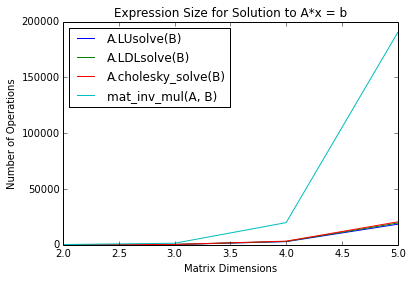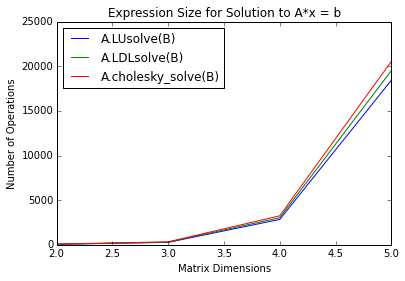Observing the above plots, once again _mat_inv_mul comes out as the worst (by far). The remaining three barely differ, but LUsolve results in the most compact expression.

# Discussion of Results¶

Overall, _mat_inv_mul comes out the worst for every benchmark, and LUsolve comes out the best. Unless I did something wrong with my benchmarks LUsolve should replace every call to _mat_inv_mul in mechanics. It results in a more compact form, and has several order of magnitude faster running speed.

This surprised me. I would have thought that a more complicated expression would take longer to solve, but as seen in the second benchmark matrix complexity had no effect on running speed for the three solution algorithms (although it did affect the substitution speed for _mat_inv_mul). I suppose that's why you're always told to profile before you optimize. Often your intuition is wrong.

Something else that surprised me was that LDLsolve and cholesky_solve had a slower running time than LUsolve. For numerical symmetric matrices, this shouldn't be the case. I wasn't able to find anything about symbolic calculation of these decompositions, but I assume it should be about the same. Either way, the more general LU decomposition seems to be the fastest.

Did I do something wrong? Disagree with these benchmarks? Let me know in the comments below!